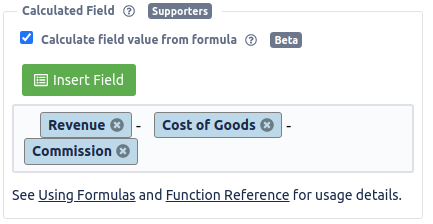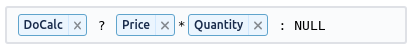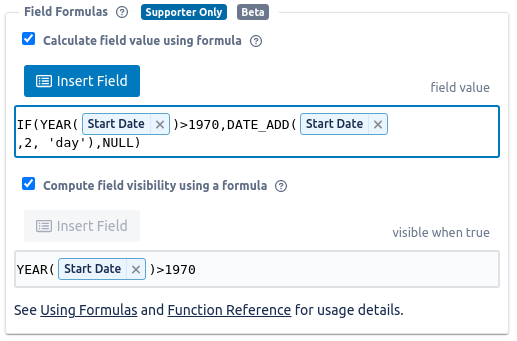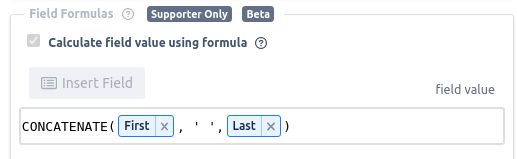# Using formulas to calculate field values

Amazing Fields for Trello lets you calculate field values using formulas just like you do in a spreadsheet. Calculate the values based upon your data inputs.

See Formulas in Action: Create a board from the Field Formula Template. Then open Amazing Fields settings to see the field details.

### Setting Up Formulas

Amazing Fields allows you to calculate the value of fields from other fields on the same Trello card.  Formulas are currently supported for Text, Number, and Checkbox field types.  Formulas are a supporter only feature so the person setting it up needs to be a supporter.  Once setup, the formula can be used by as many users and boards as you would like.

The image below shows an example formula.To setup a calculation:

• Open the Amazing Fields setting panel
• Select a field you want to compute using a formula
• Select "Calculate field value from formula" to enable the formula
• Start typing in the formula you would like
• Add references to card fields by clicking on "Insert Field"

### Using Formulas

Formulas should not contain cycles. If a field formula references other fields in a way that references the original field, the evaluation order is undefined and may lead to inconsistencies.

Once a field is set to be calculated, you can not edit it directly.  Amazing Fields will automatically update the calculation anytime you make a change to any other fields on the card.  This includes updates the user makes directly and updates made by things like linked custom fields.

If the formula fails when evaluated then Amazing Fields will popup an alert message with the details of the failure. The most common error is that the value being calculated doesn't make sense for the field type in use. Make sure to filter values and handle edge cases in your formulas.

### Supported Functions

Amazing Fields supports over 100+ of the most common functions used in spreadsheets like Excel and Google sheets.  You can see a full list in the Supported Formula Functions reference.  If you see any additional ones you would like added, please let me know.

### Default Field Values

Fields may be empty either when you create a new card or if you clear the field.  For example a numeric field like "cost" has no value on new cards.  This can cause problems for field calculations because the formulas expect all fields they use to have values.  As a result, all empty fields used in a formula will be calculated based upon a default value.  This does not actually set the field to the default value, it only adjusts the calculations behind the scenes.

The default values for each field type are:

• Number: 0.0
• Text: blank value
• Checkbox: false
• Date: 1/1/1970 00:00:00
• Dropdown:
• single select: '' (ie. empty string)
• multi-select: []  (ie. empty array)

### Clearing Field Values

There are some cases where you need to compute a value that "clears" the field to having no value.  To do this you should return the constant value `NULL` . This will tell the powerup to clear the field value.  See this example where the user has a checkbox that determines if the total price should be calculated and if it is not checked the value is cleared.### Formula Details

Formulas are evaluated using the amazing Jexl library.  The libary allows formulas that are very similar to Excel and Google Sheets but also has a few differences and extra supported operations.  For full technical details, see the Jexl page.

#### Unary Operators

Operation Symbol
Negate !

Example:  `!CompletedField`

#### Binary Operators

Operation Symbol
Subtract -
Multiply *
Divide /
Divide and floor //
Modulus %
Power of ^
Logical AND &&
Logical OR ||

Example:  `(4+5)*6`

#### Comparisons

Comparison Symbol
Equal ==
Not equal !=
Greater than >
Greater than or equal >=
Less than <
Less than or equal <=
Element in array or string in

The  `in`  operator can be used to check for a substring: `"Cad" in "Ron Cadillac"` , and it can be used to check for an array element: `"coarse" in ['fine', 'medium', 'coarse']`

Example:  `NameField == "John Snow"`

#### Ternary operator

Conditional expressions check to see if the first segment evaluates to a truthy value. If so, the consequent segment is evaluated. Otherwise, the alternate is. If the consequent section is missing, the test result itself will be used instead.

Expression Result
"" ? "Full" : "Empty" Empty
"foo" in "foobar" ? "Yes" : "No" Yes
ProgressField > 70.0 ? "Good" : "Bad" Good

#### Value Types

Type Examples
Booleans `true``false`
Strings "Hello "user"", 'Hey there!'
Numerics 6, -7.2, 5, -3.14159
Arrays ['hello', 'world!']

Note: Boolean values for true and false are `true` and `false` not `TRUE` and `FALSE` . Attempts to use the uppercase values will fail and not resolve correctly.

#### Groups

Parentheses work just how you'd expect them to:

Expression Result
(83 + 1) / 2 42
1 < 3 && (4 > 2 || 2 > 4) true

#### Constant Values

The following values may be referenced in a formula.

Identifier Description
NULL Represents an empty value. Clears the field to contain nothing.
CURRENT_VALUE The current value of the field. Useful to determine if the field has been set yet or not.

### Card Context

This is a beta capability and is not fully supported.  Please be aware that it is known to have bugs and may not be consistent in how it works.

There is beta support for accessing some card details as part of the formula.  All card values are accessed through the `card` property.

The values availabe are:

• card.desc
• card.start
• card.due
• card.dueComplete
• card.id
• card.idShort
• card.name
• card.listName
• card.url

Note: when using these values be aware that visibility and value formulas are only re-evaluated if these values change and the current user has write access to the board. (ie. if they can modify the Amazing Fields values). The code should evaluate on all changes, but there may be some small cases that are missed if the user is making a lot of edits simultaneously.

## Common Formula Usage

• Comparisons using equals (=) are not working

When comparing two values you must use double equals ( ==)instead of single equals (=).  See the list below for the full list of comparison operations.

• Using random values (RAND(), RANDBETWEEN()) updates too often

As described above, Amazing Fields updates all field formulas anytime the user edits any field on the card and when any custom field or other data causes a card to update.  When using with formulas involving random numbers this can cause some surprises because the value will keep changing.  Because of this I don't recommend using random values to determine a field output.

• Formula value is not evaluating immediately after editing

Unlike a spreadsheet, Amazing Fields formula values are not evaluated immediately.  They are only evaluated when you make a change to a value on the card.  This means that when you are editing a formula to try something new, you have to make a change on the card to force it to evaluate and compute the new value.

• Checking for empty date values

As described above, the default value of an empty date field is `1/1/1970 00:00:00` .  This makes writing logic to check for empty dates a bit confusing.  I am currently looking for a better method to handle this, but for now the easiest way to check if you have an "empty" date is to check if the `YEAR()` returns a value > 1970.  In the example below I use that technique to compute a value 2 days after the `Start Date` but only if the `Start Date` has a value.• Contatenating two text fields into a single field

This example shows how to combine the text fields for first name and last name into a combined full name.